Click to Chat

1800-1023-196

+91-120-4616500

CART 0

• 0

MY CART (5)

Use Coupon: CART20 and get 20% off on all online Study Material

ITEM
DETAILS
MRP
DISCOUNT
FINAL PRICE
Total Price: Rs.

There are no items in this cart.
Continue Shopping• Complete Physics Course - Class 11
• OFFERED PRICE: Rs. 2,968
• View Details

```The Parallel Plate Capacitor

Table of Content

Parallel Plate Capacitor

Why Parallel Plate Capacitor is used?

Dependence of Charge Stored in a Capacitor

Capacitance of Parallel Plate Capacitor

Dielectric Material inserted between two plates

Condition of parallel plate capacitor when medium is in air and in other substance

Multiplate Paralle Plate Capacitor

Charge on Parallel Plate Capacitor

Electric Field on Parallel Plate Capacitor

Parallel Plate Capacitor

Parallel Plate Capacitors are the type of capacitors which are formed by arrangement of electrodes and insulating material (dielectric)

The two conducting plates act as electrodes which are separated by a dielectric between them.

The two plates of parallel plate capacitor are of equal dimensions and is connected to power supply. The plate which is connected to positive terminal of battery acquires positive charge while the plate which is connected to negative terminal of battery acquires negative charge. Due to the attraction charges store between the plates.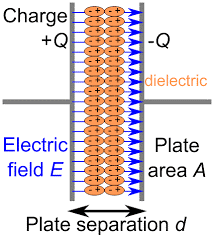Fig.1: Parallel Plate Capacitor

Why Parallel Plate Capacitor is used?

Sometimes the electric charge to be stored in a capacitor is of high amount which can’t stored in a single capacitor. So, parallel plate capacitor are used which used two plates as electrodes which help to store more electric charge.

Principle of Parallel Plate Capacitor

As we know that certain amount of charge can be given to a plate as if more charge is applied its potential will increase and charge might leak.

If we get another plate and place it next to this positively charged plate then negative charge will be pulled towards the side of this plate which is closer to the positively charged plate and positive charge on the further side.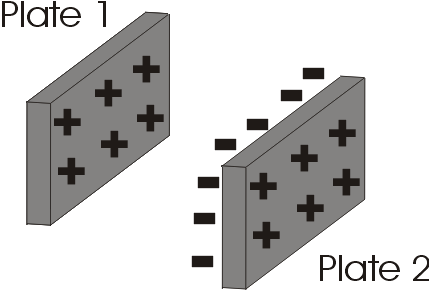Fig. 2: Two plates of parallel plate capacitor

As both the plates have charges the negative charge on plate 2 will reduce the potential difference on plate 1 while the positive charge on plate 2 will increase the potential difference on plate 1. But the negative charge on plate 2 will have more impact.so more charge can be given on plate 1 because of negative charges on plate 2 the potential difference will be less.Fig. 3: Plates of parallel plate capacitor

This is the principle of parallel plate capacitor.

Charging of Parallel Plate CapacitorFig. 4: Circuit for charging capacitor

Let C be the two plates of capacitor, V be the potential difference and k be the switch in figure 4.

Now when the key is closed then the electrons from the first plate start moving towards the positive end of the battery that is, there is flow of electrons from negative end  to positive end of the battery

The electrons which moved towards to the positive end of battery from there they will start moving towards to the second plate. In this way both the plates will acquire charges, one will acquire positive charge while other will acquire negative charge.

This process will continue until the capacitor acquires potential difference V in the exact same amount that of the battery. Now the process will stop. At this time when the process has been stopped the capacitor has stored electric charge on it with the potential difference which is same as battery.

So now the charge can be written as:

Q = CV

Dependence of Charge Stored in a Capacitor

The amount of electric charge stored in any of the plate of parallel plate capacitor is directly proportional to the potential difference between the two plates of Parallel Plate Capacitor. This relation can be seen as:

Q α  V

OR

Q = (constant) V

Q = CV

Where,

C = Capacitance of capacitor

Q = Amount of charge stored in one capacitor

V = Potential difference between the two plates

Capacitance of Parallel Plate Capacitor

The capacitance of parallel plate capacitor depends upon

The distance d between two plates

The area A of medium between the  plates

According to the gauss law, the electric field can be written as: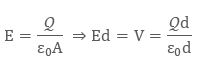Since we know that the capacitance is defined as V = Q/C, so capacitance can be rewritten as:When the plates areplaced very close and the area of plates are large we get the maximum capacitance.

Dielectric Material inserted between two plates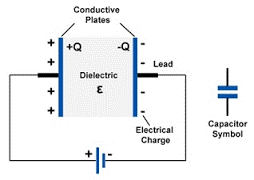Fig .5: Dielectric placed between two electrodes

On the two plates, the microscopic dipole moment of the material will shield the charges. Thus will alter the effect of dielectric material which is inserted between the two plates Materials have a permeability which is given by the relative permeability k.

The capacitance is thus given by: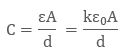Capacitance of a parallel plate capacitor can be increased by introducing dielectric between the plates as the dielectric have permeability k, which is greater than 1. K is also sometimes known as Dielectric Constant.

Condition of parallel plate capacitor when medium is in air and in other substance

When in parallel plate capacitor the area between the who plates are partially filled with air and partially with other substance its Capacitance can be calculate.

Let there exist a parallel plate capacitor in which medium between the parallel plates is mainly the air and partially other substance as shown in figure below: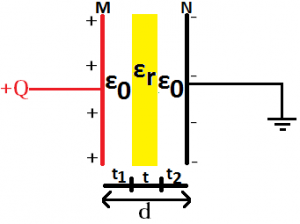Fig. 6: Dielectric and air between plates

Multiplate Paralle Plate Capacitor

The arrangement of parallel plate capacitor with dielectric material between them in groups fitting in each other is known as Multiplate Parallel Plate Capacitor.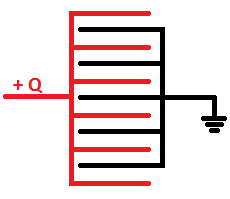Fig. 7: Multiplate Capacitor

The capacitance of multiplate parallel plate capacitor can be calculated as: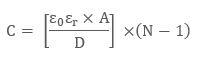where,

A = Area of each plate

ε0 = Relative Permittivity of a Vacuum = 8.854 × 10-12 F/m

εr = Relative Permittivity of Dielectric

D = Distance between plates

N = Number of Plates

Charge on Parallel Plate Capacitor

Let us assume that a capacitor has capacitance C and have electric charge Q and the capacitor is electrically neutral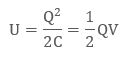where V is the potential difference between the plates.

Now if the charge upon the two plates of parallel plate capacitor are different,then

V1 will be the potential difference of plate 1 with Q1 be the charge

While V2 will be the potential difference of plate 2 with charge Q2 = −Q + δQ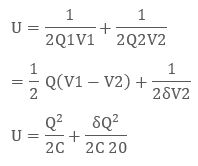Electric Field on Parallel Plate Capacitor

The electric field is assumed from both the plates of parallel plate capacitor

E =σ/2ϵ0ñ

σ is the surface charge density on a single side of the plate,

Q/2A, since half the charge will be on each side.

Parallel Plate Capacito
```### Course Features

• 101 Video Lectures
• Revision Notes
• Previous Year Papers
• Mind Map
• Study Planner
• NCERT Solutions
• Discussion Forum
• Test paper with Video Solution